# Gait Control of Hexapod Robot Based on Field-Programmable Gate Array and Central Pattern Generator

Gait Control of Hexapod Robot Based on Field-Programmable Gate Array and Central Pattern Generator
School of Microelectronics, Xidian University, Xi'an 710071, China

Corresponding Author Email:
tianboqiao@stu.xidian.edu.cn
Page:
931-937
|
DOI:
https://doi.org/10.18280/jesa.530619
3 June 2020
|
Accepted:
29 August 2020
|
Published:
23 December 2020
| Citation

OPEN ACCESS

Abstract:

This paper attempts to improve the terrain adaptability of hexapod robot through gait control. Firstly, the multi-leg coupling in the tripodal gait of the hexapod robot was modeled by Hopf oscillator. Then, annular central pattern generator (CPG) was adopted to simulate the leg movements of hexapod robot between signals. Furthermore, a physical prototype was designed for the gait control test on field-programmable gate array (FPGA), and the algorithm of the rhythmic output of the model was programmed in Verilog, a hardware description language. Finally, the effectiveness of our gait control method was verified through the simulation on Xilinx. The results show that the phase difference of the CPG network remained stable; the designed hexapod robot moved at about 5.15cm/s stably in a tripodal gait, and outperformed wheeled and tracked robots in terrain adaptation. The research findings lay a solid basis for the design of all-terrain multi-leg robots.

Keywords:

central pattern generator (CPG), hexapod robots, gait control, field-programmable gate array (FPGA)

1. Introduction

The rhythmic walking of hexapod robots is generally controlled by gait planning. The main task of gait planning is to determine the order of swing and support phases of each leg, and the sequence of continuous movement of the robot. However, the common gait planning methods have two defects: the gait planning mimics the normal rhythmic movements of natural creatures on simple terrain, failing to emulate the operations of the central nervous system in the adaptation to more complex terrains; to produce the desired gait, the stable and coordinated rhythmic signals are outputted through the contraction of muscles in the local spinal cord only.

For hexapod robots, the gait is usually controlled by kinematics and dynamics models, which integrate model simplification, stability criterion, and energy optimization [1, 2]. By these models, the robot model is firstly reduced by the stability criterion into a simple inverted pendulum. Then, the centroid of the robot and feet trajectories are determined, according to the theory of zero moment point. After that, the centroid and feet go through coordinate transform, and the trajectories of the leg joints are derived by inverse kinematics equation. Finally, the theory of zero moment point is referred to again to plan the gait of the robot, based on the actual location of the zero moment point fed back by the foot sensors. Based on the gait control, the zero moment point of the robot’s support points is controlled by adjusting the posture in the horizontal plane of the support domain, through the coordination of robot limbs [3, 4]. Then, the stable coordinates of the robot can be obtained to directly output the gait plan.

Fuzzy logic planning is a novel strategy for gait planning. The core of fuzzy logic planning is the fuzzy algorithm, a tool to describe uncertain objects. Inspired by the thinking mode of the human brain, fuzzy algorithm makes judgements by fuzzy rules to acquire the features of the object . Based on the data collected by sensors, fuzzy logic planning generates control rules between the input and output parameters of gait planning. Under these rules, the parameters of each joint are adjusted, namely, joint angle, and angular velocity . In addition, the neural network (NN) is employed to deduce the next gait from the joint trajectories and driving torque .

For vertebrates, brain consciousness is not involved in rhythmic coordinated behaviors, such as walking, breathing, and flying. In spite of that, these animals can still adapt to the changing terrain. Bionic robots have a similar gait control mechanism as vertebrates. Therefore, the neurons in the central pattern generator (CPG) of rhythmic signals are either excited or inhibited. There is no advanced control commands or external feedbacks [6-8]. Only the low-level self-excitation of the neural system is emulated. To realize coordinated movements, the robot needs to generate a stable rhythmic interlock signal through the mutual coupling between the CPG units [9, 10].

The above analysis shows that the robot movements are highly stable and flexible under the control of CPG units. The gait that ensues tends to be unstructured, and applicable only to simple terrains. If the environment is complex and unstructured, the robot movement and gait must be optimized by the cooperation between advanced and low-level controls. The former is responsible for learning and adapting to the complex terrain, and memorize the gait for the unstructured environment. In this way, the robot can move efficiently when it meets the same kind of terrain again [11, 12].

This paper mainly explores the gait control of hexapod robot based on field-programmable gate array (FPGA) and CPG. Specially, the multi-leg coupling was modeled by Hopf oscillator, and the leg movements of hexapod robot between signals were simulated by annular CPG network. Finally, a physical prototype was designed for the gait control test on FPGA. The algorithm of the rhythmic output of the model was programmed in Verilog, a hardware description language.

2. Gait Parameters

The movement pattern of footed animals is often referred to as gait, i.e., the fixed phase relationship between legs. The gait can be characterized by multiple factors, namely, the sequence of steps, step length, duty ratio, number of supporting legs, and moving speed.

The sequence of steps refers to the order of movement between legs. By adjusting the sequence and frequency of steps, it is easy to change the body position and posture.

The step length is also known as stride length. When one leg is in the swing phase (the process of a leg swinging forward during the gait cycle), the distance between the foot and the landing spot is half the step length. Likewise, the process of a leg supporting the body during the gait cycle is called stance phase .

The duty ratio is defined as the time fraction of stance phase in a gait cycle :

$\beta=\frac{t_{\text {stance}}}{T}=1-\frac{t_{\text {swing}}}{T}$  (1)

where, tstance is the time of stance phase in a gait cycle; tswing is the time of swing phase in a gait cycle; T is the gait cycle:

$T=t_{\text {stance}}+t_{\text {swing}}$   (2)

Let n be the number of legs. Then, the number of supporting legs can be calculated by:

$\delta=\mathrm{n} \times \beta$  (3)

The moving speed v can be defined as:

$V=\frac{s}{t_{stance}}$  (4)

where, s is the step length. From formulas (1)-(4), we have:

$v=\frac{s}{t_{\text {swing }}}\left(\frac{1}{\beta}-1\right)$  (5)

In a normal gait, the duty ratio is negatively correlated with the moving speed. This is because the step length and the time of swing phase of each leg are generally invariant, similar to the walking features of insects in nature .

For bionic robots, the time of swing phase usually remains constant. For each leg, the greater the duty ratio, the longer the stance phase, and the slower the movement . If the duty ratio is increased, more legs will support the body, making the body more stable.

3. CPG-Based Gait Control

Hopf oscillator is a class of nonlinear coupled oscillators . Based on the coupling between nerve axons, Hopf oscillator can be easily expressed mathematically. Moreover, the parameters of this oscillator can be adjusted at ease. The mathematical expression of Hopf oscillator can be defined as:

$\left\{\begin{array}{l}\dot{x}=\lambda\left(K-r^{2}\right) x-\omega y \\ \dot{y}=\lambda\left(K-r^{2}\right) y-\omega x \\ r=x^{2}+y^{2}\end{array}\right.$   (6)

where, K is the correction factor of the amplitude ($A=\sqrt{K}, K>0$) of the signal generated by the coupling of Hopf nerve axons; $\omega$ is the frequency of the oscillator; $x, y$ are the output variables of the coupling.

Thanks to its convergence nature, the Hopf oscillator tends to output a sine or cosine curve in a certain time range. The specific waveform depends on the number of initial coupling state variables. The phase trajectories of these variables also exhibit a sine or cosine pattern.

Let $\lambda$ be the speed of the signal to reach the stable speed. Under the conditions of $\lambda=500, \omega=2 \pi$, and $A=2$, the limit cycle and output curve of the Hopf oscillator are displayed in Figure 1.

## 1a.jpg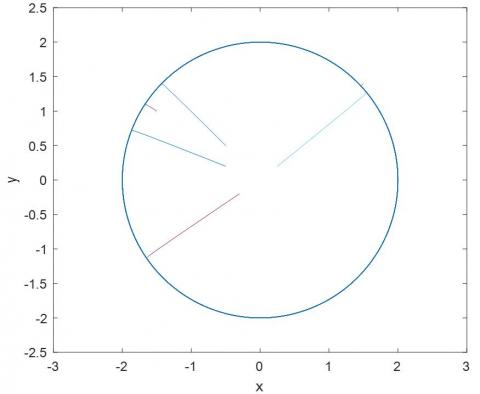## 1b.jpg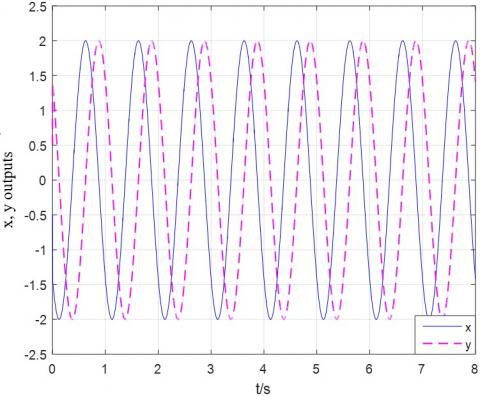Figure 1. The (a)limit cycle and (b)output curve of Hopf oscillator

4. Typical Gaits

4.1 Phase relationship of each gait

The gait of six-legged insects (e.g., ant and ladybug) are usually described by gait diagrams like zebra lines. The three most common gaits are tripodal gait (Figure 2), quadrupedal gait (Figure 3), and fluctuating gait. The main difference between these gait lies in the number of supporting legs.

## 2.jpg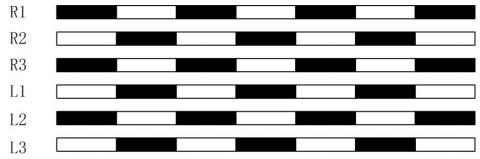Figure 2. The sequence of steps in tripodal gait

## 3.pngFigure 3. The sequence of steps in quadruped gait

Tripodal gait is the fastest gait of six-legged insects during steady walking. The duty ratio of this gait is slightly above 0.5. In each step, three legs (R2, L1, and L3) touch the ground, forming a stable triangular support structure. Meanwhile, the other three legs (R1, R3, and L2) swing forward rapidly toward the landing spot. After landing, a new triangular support structure is formed. This is the defining feature of tripodal gait. The six-legged insect repeats the above process to move forward.

Covering only swing and stance phases, the tripodal gait is simple to implement. Therefore, this gait has been widely used in bionic robots with simple control systems. The phase relationship is summarized below:

(1) L1, L3, and R2 are in phase;

(2) L2, R1, and R3 are in phase;

(3) (L1, L3, R2) phase is ahead of (L2, R1, R3)*T/2.

The quadrupedal gait is a relatively slow gait with four legs on the ground at any moment. Meanwhile, one leg on each side swings forward. This gait can withstand a large load, thanks to its long support time. The phase relationship is summarized below:

(1) L1 and R1 are in phase;

(2) L2 and R2 are in phase;

(3) L3 and R3 are in phase;

(4) (L1,R1) phase is ahead of (L2,R2)T/3;

(5) (L2,R2) phase is ahead of (L3,R3)T/3.

For bionic robots, the gait changes depend on the speed and load of movements. The heavier the load, more supporting legs are needed per unit time. That is, the greater the number of supporting legs and duty ratio, the longer the time of stance phase in a gait cycle, and the slower the moving speed.

4.2 Phase relationship of each leg

As shown in Figure 4, during the movements of hexapod robot, the legs on each side lift and drop in sequence from front to back. The back legs repeat the movements of the front legs in turn. Overall, the leg movements spread like a wave.

To evaluate the phase relationship of movement propagation between legs, the concept of beat was defined as the time when a movement of a leg spread to the next leg . The phase difference between adjacent legs on the same side is clearly one beat.

Next, the synchronization between the movements of each leg in two most typical gaits of bionic robots are plotted in Figure 5, aiming to illustrate the phase difference between the gait waves of the legs on both sides. The synchronization in the tripodal gait is presented on the left, and that in the quadrupedal gait is given on the right. It can be seen that the right leg is one beat behind the left in the tripodal gait, and two beats behind in the quadrupedal gait. Hence, for an N-feet gait, the right leg must be (N-2) beats behind the left leg.

When the hexapod robot is half occupied, it moves forward in the tripodal gait . In this case, the robot legs take the same amount of time in swing phase as in support phase. In other words, the ascending and descending curves of CPG output signals have the same duration. To realize stable control of the robot, the output signals were divided into rhythmic signals of leg movements with significant phase in one gait cycle.

## 4.pngFigure 4. The sequence of leg movements

## 5.pngFigure 5. The synchronization between legs

4.3 Coupling design of leg joints

During the movement of the hexapod robot, the hip and knee joints often obey a certain law of movement, which depends on the hip joint coordination between the six legs. The legs belong to different groups in terms of phase angle difference. To ensure the degrees of freedom, the rotation of each leg joint must be realized by the coupling between the joints in the leg. Otherwise, the body and legs cannot move stably in coordination .

To map the oscillator output signal x, y and the rhythmic signal regulating the hip and knee movements, the two signals are compared in Figure 6. In actual movement, the hexapod robot needs to adjust its movement to adapt to the complex terrain. Thus, the movement time of the supporting leg might differ from that of the swinging leg . Therefore, the original Hopf oscillator was improved to control the support phase and the swing phase separately. The original Hopf oscillator can be expressed as:

$\left\{\begin{array}{l}\omega=\frac{\omega_{S t}}{e^{-b y_{+1}}}+\frac{\omega_{S W}}{e^{b y_{+1}}} \\ \omega_{s t}=\frac{1-\eta}{\eta} \omega_{S W}\end{array}\right.$  (7)

where, $\omega_{S W}$ and $\omega_{s t}$ are determinants of the duration of the swing phase, and the support phase, respectively; $b$ is the time ratio between the two phases; $\eta$ is the duty ratio.

Adding the external feedbacks f1 and f2 to formula (7), the improved Hopf oscillator can be expressed as:

$\left\{\begin{array}{l}\dot{x}=\lambda\left(K-r^{2}\right)\left(x-f_{1}\right)-\omega\left(y-f_{2}\right) \\ \dot{y}=\lambda\left(K-r^{2}\right)\left(y-f_{2}\right)-\omega\left(x-f_{1}\right) \\ r=\left(x-f_{1}\right)^{2}+\left(y-f_{2}\right)^{2} \\ \omega=\frac{\omega_{s t}}{e^{-b y}+1}+\frac{\omega_{s w}}{e^{b y}+1} \\ \omega_{s t}=\frac{1-\eta}{\eta} \omega_{s w}\end{array}\right.$   (8)

## 6.pngFigure 6. The regular leg movements of hexapod robot

5. Simulation

5.1 3D Model

The target hexapod robot is approximately a symmetry rectangle. Among the numerous gaits of the robot, the most typical gait is tripodal gait. It is assumed that the centroid of the robot is the geometric center of the rectangle. During the simulation analysis, the feet in the supporting leg and the swinging leg are illustrated as solid dots and hollow dots, respectively.

Before three-dimensional (3D) modeling in ADAMS, the following simplifications were conducted: (1)The body of the hexapod robot was simplified as a rectangular box with uniform density to maintain the relationship between the body and the hip joint; (2) The hip joint, knee joint, and ankle joint of each leg were regarded as nodes in a connecting rod system to maintain the relative positions between them in the leg; (3) The connections between the three joints and the body was treated like a rotating pair.

After the simplifications, the horizontal ground was simulated in the following steps: First, build a cuboid on the bottom surface to emulate the horizontal ground, and set up constraints between the feet and the horizontal plane; Second, set the material of the body as aluminum alloy, and automatically generate the centroid and other parameters of each component in ADAMS; Third, set the direction of gravity vertically downward with the default value of 9.85. Through the above steps, the 3D modeling of the hexapod robot is successful if “Model Successfully Verified” appears in the dialog box.

5.2 Control system

The established 3D model was imported to MATLAB to obtain the input and output variables. Then, the CPG network was established mathematically based on the system module of ADAMS. The block diagram of the CPG control system is presented in Figure 7. The structure of a single neuron oscillation is explained in Figure 8. The entire control system consists of six subsystems, each of which could generate a set of changeable waveforms. In total, the control system can generate 18 waveforms to meet the requirements of the tripodal gait of the hexapod robot.

## 7.jpgFigure 7. The block diagram of the CPG control system

## 8.jpgFigure 8. The structure of single neuron oscillation

Next, the simulation time was set to 5s, and a MATLAB simulation was conducted on the control system. Figure 9 shows the dynamic movements of the hexapod robot in tripodal gait from 0-1.5s.

## 9.jpg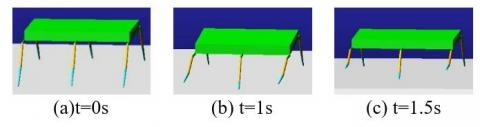Figure 9. The dynamic movements of the hexapod robot in tripodal gait

5.3 FPGA hardware board

LMD18200 is a 3A H-bridge specially designed for movement control applications. Through multi-functional design, this device integrates bipolar and CMOS (complementary metal oxide semiconductor) control circuits with DMOS (double diffused metal oxide semiconductor) power devices into the same monolithic structure. It provides an ideal driver for direct current (DC) and the driver chip of stepping motor. LMD18200 can accommodate up to 6A peak output current, and drive 10-30V motors . Figure 10 illustrates the circuit diagram of LMD18200.

## 10.jpgFigure 10. The circuit diagram of LMD18200

Four DMOS tubes can be integrated in LMD18200 to form a standard H-type drive bridge. The two switch tubes of the upper bridge arm are provided with a gate control voltage by the charge pump circuit, which is always maintained at the operating frequency around 300kHZ. When the armature of the DC motor is connected to pins 2 and 10, the current will flow from pin 2 to pin 10 in forward rotation, and backward in reverse rotation. If overcurrent lasts for a long time, the overheat protector will shut down the entire current output, and pin 9 will output an overheat signal [21, 22].

This design relies on pulse width modulation (PWM) to control analog circuits with digital signals. After the opening and closing of power tubes are controlled, the DC voltage can be converted into a series of fixed-frequency pulse signals through the PWM. By changing the duty cycle of these pulse signals, the mean output voltage can be changed, thereby regulating the motor speed. The PWM converts analog circuits into digital signals from the controller to the controlled system, eliminating the need for digital-to-analog conversion. This saves lots of cost and space, and improves the anti-interference ability of the controller.

After the motor is powered on, the motor speed increases at the arrival of a pulse; After the motor is powered off, the motor speed slows down. The motor speed can be automatically controlled. Let $V_{\max }$ be the maximum motor speed, and $D=\frac{t_{1}}{T}$ be the duty cycle, when the power supply is uninterrupted. Then, the mean motor speed $V_{d}$ can be calculated by:

$V_{d}=V_{\max }$  (9)

Formula (9) shows that the mean motor speed is proportional to the duty cycle, and peaks at the duty cycle of 1.

In the circuit diagram, there are two counters, two NOT gates, and a D flip-flop. Counters 1 and 2 are the on and off switches, respectively. Both counters can be set in advance to desired numbers. Since counter output is actually the number of clock signals, the turn-on or turn-off time is the clock period multiplied by the number of counts. The circuit is realized by the following principles:

At the start of the circuit, Q=0, counter 2 waits for counting, and counts clock from 0, and C2=0; counter 1 is preset to the number Don; when counter 2 counts from 0 to 49, C2=1 is outputted, and D flip-flop output is set to Q=1.

At Q=1, counter 1 starts to wait for counting, and counts clock from 0, and C1=0; counter 2 is preset to the number Doff; when counter 1 counts from 0 to 49, C1=1 is outputted, and D flip-flop output is set to Q=0.

At Q=0, counter 2 starts counting from the preset number Doff to 49, and then outputs C2=0; counter 1 is preset to the desired number; when counter 2 counts from the preset number to 49, C2=1 is outputted, and D flip-flop output is set to Q=1.

The duty cycle of the output signal in the hardware circuit can be calculated by:

$out=\frac{50-\text { Don }}{[(50- { Don })+(50- { Dof } f)]}=\frac{50- { Don }}{100- { Don }- { Dof } f}$   (10)

The voltage was regulated through fixed-frequency PWM. To change the size of the duty cycle,

Xilinx FPGA was employed, and the PWM module was written in Verilog. By writing the code of the counter module, the PWM waveform was generated with fixed pulse period. The two modules worked together to realize the duty cycle.

The task logic of the PWM module consists of the system input clock clk, reset signal reset, preset number Don, preset number Doff, and PWM output signal PWM.

5.4 Debugging and result analysis

The PWM digital signals were generated based on the Artix-7 XC7A100T FPGA (Xilinx), and Verilog programming in the ISE Design Suite. The signals thus obtained feature simple structure, easy modification, precise control, and flexible use. This FPGA processing chip greatly simplifies the system structure and parameter modification, while improving the stability of PWM signals.

Without exceeding the FPGA resources, the duty cycle of the PWM signal could be set arbitrarily without affecting the accuracy of the final result. The rhythmic signal code was written in Verilog, and the duty ratio was set to 50% to obtain the final simulation program.

Figure 11 records the time variation in the movements of robot centroid. It can be seen that the centroid of the robot shifted by about 3m within 5s, but did not fluctuate significantly throughout the movement. This means the robot could move stably.

Figure 12 displays the movements of each joint in the hexapod robot. Obviously, the support and swing phases of the robot alternatively appeared, completing coordinated and stable movements in the tripodal gait.

Figure 13 shows the simulation waveforms at Don=45 and Doff=45, and the duty cycle is 50%. the simulation waveform of input preset number Don=25, Doff=35, the duty ratio is 62.5%. It can be seen that: (1) The robot joints output a coordinated rhythmic signal; when the leg curve rose, the corresponding leg was in the swing phase; when the leg curve dropped, the corresponding leg was in the support phase. (2) The phase difference between leg groups in the hip joint output curve, denoted as π, amounts to half a cycle, such that different leg groups took turns to complete coordinated movement; the hip joint control signals were the same among legs in the same group.

## 11.jpg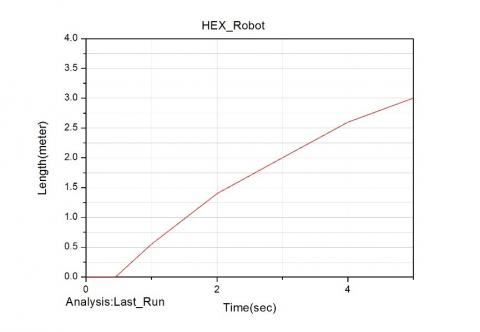Figure 11. The time variation in the movements of robot centroid

## 12a.jpg(a) Angular velocity of each joint in the left foreleg

## 12b.jpg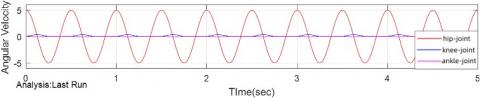(b) Angular velocity of each joint in the right foreleg

Figure 12. The movements of each joint in the hexapod robot

## 13.jpgFigure 13. The simulation waveforms at Don=45 and Doff=45

6. Conclusions

Multi-leg robots are widely recognized for their flexibility and terrain adaptability. To realize coordinated stable movements of hexapod robot, it is of practical significance to simulate the movement control mode of CPG. From the perspective of robot gait planning, this paper simulates and controls the coordinated and smooth movements of hexapod robot, using the rhythmic signal generated by the neural oscillation tissue of CPG. Firstly, the CPG control principles of footed animal movements were analyzed to derive the control method for gait generation and transform during robot movements. Next, the Hopf oscillator was improved to keep the duration of supporting phase equal to that of swinging phase of the robot. Further, the CPG was introduced to realize the coordinated control of multiple joints between and within the feet of hexapod robot. Based on the structure of the Hopf oscillator, a leg joint control module was established, consisting of six oscillators in a hierarchical topology. The proposed method was verified through Xilinx simulation. The results show that our gait control strategy can effectively regulate various typical gaits of hexapod robot, and meet the load requirements for the gait control of the robot’s rhythmic movements. In addition, the relevant control module is easy to build, flexible to adjust, and convenient for real-time control.

References

 Bal, C. (2021). Neural coupled central pattern generator based smooth gait transition of a biomimetic hexapod robot. Neurocomputing, 420: 210-226. https://doi.org/10.1016/j.neucom.2020.07.114

 Barron-Zambrano, J.H., Torres-Huitzil, C., Girau, B. (2015). Perception-driven adaptive CPG-based locomotion for hexapod robots. Neurocomputing, 170: 63-78. https://doi.org/10.1016/j.neucom.2015.02.087

 Rostro-Gonzalez, H., Cerna-Garcia, P.A., Trejo-Caballero, G., Garcia-Capulin, C.H., Ibarra-Manzano, M.A., Avina-Cervantes, J.G., Torres-Huitzil, C. (2015). A CPG system based on spiking neurons for hexapod robot locomotion. Neurocomputing, 170: 47-54. https://doi.org/10.1016/j.neucom.2015.03.090

 Ijspeert, A.J. (2008). Central pattern generators for locomotion control in animals and robots: a review. Neural Networks, 21(4): 642-653. https://doi.org/10.1016/j.neunet.2008.03.014

 Gutierrez-Galan, D., Dominguez-Morales, J.P., Perez-Peña, F., Jimenez-Fernandez, A., Linares-Barranco, A. (2020). NeuroPod: A real-time neuromorphic spiking CPG applied to robotics. Neurocomputing, 381: 10-19. https://doi.org/10.1016/j.neucom.2019.11.007

 Liu, C., Li, Z., Zhang, C., Yan, Y., Zhang, R. (2019). Gait planning and control for a hexapod robot on uneven terrain based on Markov decision process. 2019 14th IEEE Conference on Industrial Electronics and Applications (ICIEA), Xi'an, China, pp. 583-586. https://doi.org/10.1109/ICIEA.2019.8834181

 Bai, L., Hu, H., Chen, X., Sun, Y., Ma, C., Zhong, Y. (2019). CPG-based gait generation of the curved-leg hexapod robot with smooth gait transition. Sensors, 19(17): 3705. https://doi.org/10.3390/s19173705

 Carvalho, C.S., Raptopoulos, L.S., Andrade, W.S., Alves, D.S., Dutra, M.S., França, F.M. (2015). Extending SMER-based CPGs to accommodate total support phases and kinematics-safe transitions between gait rhythms of hexapod robots. Neurocomputing, 170: 113-127. https://doi.org/10.1016/j.neucom.2015.04.091

 Inada, H., Ishii, K. (2004). Bipedal walk using a central pattern generator. International Congress Series, 1269: 185-188. https://doi.org/10.1016/j.ics.2004.05.129

 Chen, Q., Wang, J., Yang, S., Qin, Y., Deng, B., Wei, X. (2017). A real-time FPGA implementation of a biologically inspired central pattern generator network. Neurocomputing, 244: 63-80. https://doi.org/10.1016/j.neucom.2017.03.028

 Chen, Y., Liu, S.S. (2015). Fourfold frequency multiplication circuit design of photoelectric encoder based on CPLD. Foreign Electronic Measurement Technology, (1): 45-48.

 Barron-Zambrano, J.H., Torres-Huitzil, C. (2013). FPGA implementation of a configurable neuromorphic CPG-based locomotion controller. Neural Networks, 45: 50-61. https://doi.org/10.1016/j.neunet.2013.04.005

 Lei, Z., Xin, D., Hua, J. (2017). Motion simulation of bionic hexapod robot based on virtual prototyping technology. 2017 International Conference on Computer Network, Electronic and Automation (ICCNEA), Xi'an, pp. 426-430. https://doi.org/10.1109/ICCNEA.2017.28

 Inagaki, S., Yuasa, H., Arai, T. (2003). CPG model for autonomous decentralized multi-legged robot system-generation and transition of oscillation patterns and dynamics of oscillators. Robotics and Autonomous Systems, 44(3-4): 171-179. https://doi.org/10.1016/S0921-8890(03)00067-8

 Raibert, M., Blankespoor, K., Nelson, G., Playter, R. (2008). Bigdog, the rough-terrain quadruped robot. IFAC Proceedings Volumes, 41(2): 10822-10825. https://doi.org/10.3182/20080706-5-KR-1001.01833

 Buehler, M., Playter, R., Raibert, M. (2005). Robots step outside. In Int. Symp. Adaptive Motion of Animals and Machines (AMAM), Ilmenau, Germany, pp. 1-4.

 Xiao, Q., Qin, M., Yin, Y. (2020). Skeleton-based Chinese sign language recognition and generation for bidirectional communication between deaf and hearing people. Neural Networks, 125: 41-55. https://doi.org/10.1016/j.neunet.2020.01.030

 Yu, H., Gao, H., Deng, Z. (2020). Enhancing adaptability with local reactive behaviors for hexapod walking robot via sensory feedback integrated central pattern generator. Robotics and Autonomous Systems, 124: 103401. https://doi.org/10.1016/j.robot.2019.103401

 Brooks, R.A. (1989). A robot that walks; emergent behaviors from a carefully evolved network. Neural Computation, 1(2): 253-262. https://doi.org/10.1162/neco.1989.1.2.253

 Barrio, R., Lozano, Á., Rodríguez, M., Serrano, S. (2020). Numerical detection of patterns in CPGs: Gait patterns in insect movement. Communications in Nonlinear Science and Numerical Simulation, 82: 105047. https://doi.org/10.1016/j.cnsns.2019.105047

 Hao, X., Yang, S., Deng, B., Wang, J., Wei, X., Che, Y. (2021). A CORDIC based real-time implementation and analysis of a respiratory central pattern generator. Neurocomputing, 423: 373-388. https://doi.org/10.1016/j.neucom.2020.10.101

 Yu, Q., Wang, B., Zhong, R., Huang, L., Gu, Z., Zhang, T., Huang, G., Hou, W., Jin, Z. (2020). Visualization system of orthopedic personalized orthosis based on FPGA and sensor. Microprocessors and Microsystems, 103581. https://doi.org/10.1016/j.micpro.2020.103581